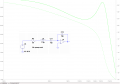# Why is the resonance frequency of RLC with OpAmp not the same as without OpAmp?

#### Matjelo Naleli

Joined Oct 7, 2015
42I have LTSpice simulation of RLC plus OpAmp circuit as shown in the diagram. I was aiming to build a transfer function (voltage to voltage) of the following kind:

G(s) = -1/(L*C*s^2 + R*C*s + 1)

with resonance frequency at
f= 1/(2*pi*(L*C)^(1/2)) = 1/(2*pi*(7.5uH*15pF)^(1/2)) = 15MHz

However I get resonance at around 20MHz.

What could be the problem with my implementation which leads to 20MHz instead of 15MHz?

Thanks

#### Jony130

Joined Feb 17, 2009
5,445
You must increase the op amp GBP. But nevertheless the circuit will not work in real life.

#### MikeML

Joined Oct 2, 2009
5,444
Last edited:
•Matjelo Naleli

#### bertus

Joined Apr 5, 2008
22,138
Hello,

The about 20 Mhz is as the two capacitors in series are seen.
Then you have a total capacity of 7.5 pF, this results in a frequency of 21.22 Mhz.
This happens because the input impedance of the opamp in infinite and the output impedance is 0.

Bertus

•Matjelo Naleli

#### MikeML

Joined Oct 2, 2009
5,444
Hello,

The about 20 Mhz is as the two capacitors in series are seen.
Then you have a total capacity of 7.5 pF, this results in a frequency of 21.22 Mhz.
This happens because the input impedance of the opamp in infinite and the output impedance is 0.

Bertus
With an ideal opamp, there is a virtual ground between the two capacitors. The current that flows through the series RLC to the left of the virtual gnd also flows in the feedback capacitor with a negative output, and the resonance is determined only by the by 15pF.

Only if the opamp is non-ideal, and the virtual gnd isn't, (e.g. too low GBP) would the resonance shift higher in frequency...

•Matjelo Naleli

#### bertus

Joined Apr 5, 2008
22,138
Hello,

OK, I am wrong. Glad you corrected the matter.
The frequency I found is like there are the two capacitors in series.

Bertus

#### Matjelo Naleli

Joined Oct 7, 2015
42
Oh great I didn't know that the two capacitors are can be taken to be in series given tha there is ground between them. I naively took the transfer function to be the ratio of the feedback capacitor impedance and the input series RLC impedance.
Thanks bertus for pointing that out, I am not sure if I understand how they are in series but the resulting frequency seems to be indicative of the serial connection of the two capacitors.

MikeML, the frequency response that you showed there is exactly what I was hoping to get. So does this mean the opamp that I used is not ideal while the dependent voltage source you used can be seen as an ideal opamp? Also is 1e5 the gain of the voltage dependent voltage source?

#### MikeML

Joined Oct 2, 2009
5,444
If the opamp has more than an open-loop gain of ~100 at 20MHz, the two capacitors are not "in-series" for the purpose of determining the poles of the transfer function. Read post #5 again, and Bertus's response in #6.

gain = 1e5 is typical for most real opamps. The voltage-controlled voltage source wired as I show it behaves like an ideal opamp with infinite input impedance, zero bias current, zero output impedance, and most importantly for your case, infinite gain-bandwidth product, but a specified open loop gain...

#### Matjelo Naleli

Joined Oct 7, 2015
42
Whenever I work with RC networks plus opamps I get the resonance frequency where it's supposed to be. But with RC networks it's not easy to get 15MHz without using small resistors which overload the opamp. So I then decide to include inductors and that's when I get this frequency shift all the time. At some point I ended up thinking that the inductor is incompatible with opamps.
But now I think Jony130 and MikeML have pointed out something that didn't cross my mind, that of GBP or non-idealness of the opamp.